# 2D_3D_Geometry

## 1. 2D/3D points, poses and homogeneous coordinates

Using Homogeneous coordinates is one way of composing 2D or 3D translations and rotations easily. This page describes a set a set of classes in the MRPT C++ library aimed for 2D/3D geometry computations, which internally rely on these matrices.

## 2. Spatial geometry classes in the MRPT

The base class for all 2D/3D point and pose classes is poses::CPoseOrPoint (see class hierarchy graph).

This class and those directly derived from it (CPose and CPoint) are abstract so you cannot directly create instances of them. Instead, the classes a user should always employ are CPoint2D, CPoint3D, CPose2D, CPose3D and CPose3DQuat, described next.

Note that these classes only represent one value, whereas another set of classes (see this page) describes probability density functions over geometry relations.

### 2.1. CPoint2D: (x,y)

This class represents a 2D point (z coordinates defaults to 0):

 Homogeneous transfomation matrix Spatial representation$\mathbf{R} = \left( \begin{array}{cccc} 1 & 0 & 0 & x \\ 0 & 1 & 0 & y \\ 0 & 0 & 1 & 0 \\ 0 & 0 & 0 & 1 \end{array} \right)$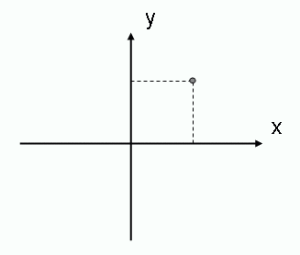An example of a construction of such an object with some initial values:

### 2.2. CPoint3D: (x,y,z)

This class represents a 3D point:

 Homogeneous transfomation matrix Spatial representation$\mathbf{R}=\left( \begin{array}{cccc} 1 & 0 & 0 & x \\ 0 & 1 & 0 & y \\ 0 & 0 & 1 & z \\ 0 & 0 & 0 & 1 \end{array} \right)$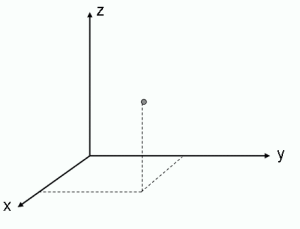An example of a construction of such an object with some initial values:

### 2.3. CPose2D: (x,y,ϕ)

This class represents a 2D location plus a heading angle (also called ”yaw” or ”orientation”). This is the typical representation of ground robots working on flat scenarios. The z coordinate defaults to 0.

 Homogeneous transfomation matrix Spatial representation$\mathbf{R}=\left( \begin{array}{cccc} \cos\phi & -\sin\phi & 0 & x \\ \sin\phi & \cos\phi & 0 & y \\ 0 & 0 & 1 & 0 \\ 0 & 0 & 0 & 1 \end{array} \right)$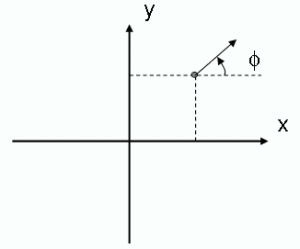An example of a construction of such an object with some initial values:

### 2.4. CPose3D: (x,y,z,yaw,pitch,roll)

This class represents a full 6D pose: a 3D position plus three attitude angles: yaw, pitch, and roll, defined as can be seen in the figure (note positive pitch angles lie below the XY plane):

 Homogeneous transfomation matrix Spatial representation$\mathbf{R}=\left( \begin{array}{cccc} cycp & cyspsr-sycr & cyspcr+sysr & x \\ sycp & syspsr+cycr & syspcr-cysr & y \\ -sp & cpsr & cpcr & z \\ 0 & 0 & 0 & 1 \end{array} \right)$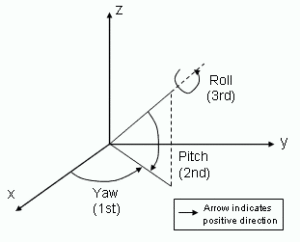The abbreviations employed in the matrix are:$\begin{array}{ll} cy = \cos yaw & sy = \sin yaw \\ cp = \cos pitch & sp = \sin pitch \\ cr = \cos roll & sr = \sin roll \end{array}$

An example of a construction of such an object with some initial values:

### 2.5. CPose3DQuat: 3D translation + quaternion (x,y,z, qr,qx,qy,qz)

This class represents a full 6D pose: a 3D position (x,y,z) plus a quaternion for the 3D rotation (qr,qx,qy,qz):$\begin{pmatrix} a^2+b^2-c^2-d^2&2bc-2ad &2bd+2ac \\ 2bc+2ad &a^2-b^2+c^2-d^2&2cd-2ab \\ 2bd-2ac &2cd+2ab &a^2-b^2-c^2+d^2\\ \end{pmatrix}$

An example of a construction of such an object:

## 3. Derivation of the 3D rotation matrix

The 3×3 transformation matrix (also called Direction Cosine Matrix, DCM) for an arbitrary combination of yaw, pitch and roll rotations can be derived from the
combination of the individual rotations:$R_z R_y R_x = \left( \begin{array}{ccc} \cos yaw \cos pitch & \cos yaw \sin pitch \sin roll - \sin yaw \cos roll & \cos yaw \sin pitch \cos roll + \sin yaw \sin roll \\ \sin yaw \cos pitch & \sin yaw \sin pitch \sin roll + \cos yaw \cos roll & \sin yaw \sin pitch \cos roll - \cos yaw \sin roll \\ -\sin pitch & \cos pitch \sin roll & \cos pitch \cos roll \end{array} \right)$

with:$R_x = \left( \begin{array}{ccc} 1 & 0 & 0 \\ 0 & \cos roll & -\sin roll \\ 0 & \sin roll & \cos roll \end{array} \right)$$R_y = \left( \begin{array}{ccc} \cos pitch & 0 & \sin pitch \\ 0 & 1 & 0 \\ -\sin pitch & 0 & \cos pitch \end{array} \right)$$R_z = \left( \begin{array}{ccc} \cos yaw & -\sin yaw & 0 \\ \sin yaw & \cos yaw & 0 \\ 0 & 0 & 1 \end{array} \right)$

Here we have used the fact that rotations over successively transformed axes are achieved by right-side matrix multiplications,
i.e. the order of the rotations is Rz, then Ry, then Rx. For more information about the order of matrix multiplications, see Euler angles -> DCM on Wikipedia.

More conventions are discussed in http://mathworld.wolfram.com/EulerAngles.html

## 4. Conversions between classes

Objects of any pose or point class can be assigned to any other, and the conversion will preserve all the information as possible. Most of the conversions can be made implicitly, as in:

But for conversions from classes with more information than the target class, they must be written explicitly by the user, as in the example below. This rule (introduced in MRPT 0.6.5) tries to avoid unintentional losses of information.

## 5. Common operators

### 5.1. Pose composition / inverse composition

Pose composition is the process of changing the coordinate system reference of some given point or pose B to that described by another point or pose A, obtaining a new pose or point C. Using MRPT C++ library classes this process can be implemented as simply as:

The operation will naturally take into account all the required rotations (through homogeneous matrix multiplication). The inverse process, obtaining the relative coordinates B of a point with global coordinates C as seen from another reference A, is accomplished by the – operator:

Points, poses, 2D, and 3D can be all freely mixed in these operations. The following tables summarize the expected output from all the input combinations:

Does “a+b” return a Pose or a Point?

 a \ b Pose Point Pose Pose Point Point Pose Point

Does “a-b” return a Pose or a Point?

 a \ b Pose Point Pose Pose Pose Point Point Point

Does “a+b” (and “a-b”) return a 2D or a 3D object?

 a \ b 2D 3D 2D 2D 3D 3D 3D 3D

### 5.2. Distances

There are a few distance-related methods applicable to any point or pose:

• Distance from this object to any other point or pose (for a 2D object it will take into account Z coordinates if compared to a 3D object):
• Square of the distance from this object to any other point or pose:
• Distance from this object to any user supplied 2D or 3D coordinates:
• And their square distance equivalents:
Check the doxygen reference documentation of poses::CPoseOrPoint for more details.

### 5.3. Norm

The method:

Returns the Euclidean norm of the point:$||\mathbf{x}|| = \sqrt{x^2+y^2+z^2}$

### 5.4. Output to console

All the point/pose classes define a convenient operator

## 6. Quaternions

An alternative representation of 3D rotations are quaternions. MRPT supports them through the class:

template mrpt::math::CQuaternion

There exist methods to convert from CPose3D‘s and quaternions, but note that this only holds for pure rotations (without translations).
For complete 6D poses with quaternions, see the class CPose3DQuat.

## 7. An example: A mobile robot sensing with a camera

This example considers a mobile robot with a known 2D pose (x,y,heading) R. It has a camera onboard, with a relative 6D pose C relative to the robot reference. We will compute the 3D position (x,y,z) of a global landmark as seen by the camera, where L and L’ will stand for the absolute and camera-relative coordinates of this landmark. The scenario is depicted in the figure: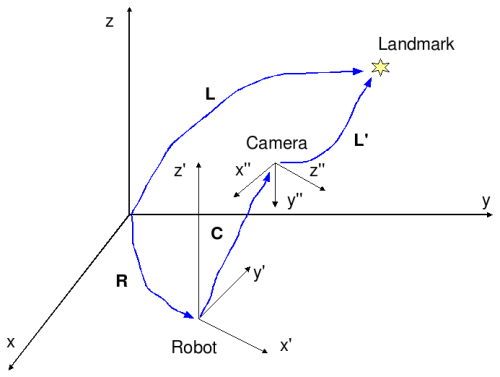Then, the landmark as observed by the camera is at:$\mathbf{L}' = \mathbf{L} \ominus ( \mathbf{R} \oplus \mathbf{C} )$

Next, we’ll check the correctness of the calculations by projecting this observation from the camera, which must coincide with the original absolute coordinates:$\mathbf{R} \oplus \mathbf{C} \oplus \mathbf{L'} \doteq \mathbf{L}$

As an example of the pose constructors, consider the camera pose relative to the robot:

The first three arguments are the x,y,z coordinates of the camera. The rest are yaw=-90º, pitch=0º, and roll=-90º. This generates the common configuration of the +Z axis of the camera pointing forward (refer to the figure).

The corresponding source code for the whole example is listed next (also found in MRPT/samples/geometry3D/test.cpp):

[spoiler style=”2″ title=”See C++ source”] [/spoiler]

And this is the output generated by the program: